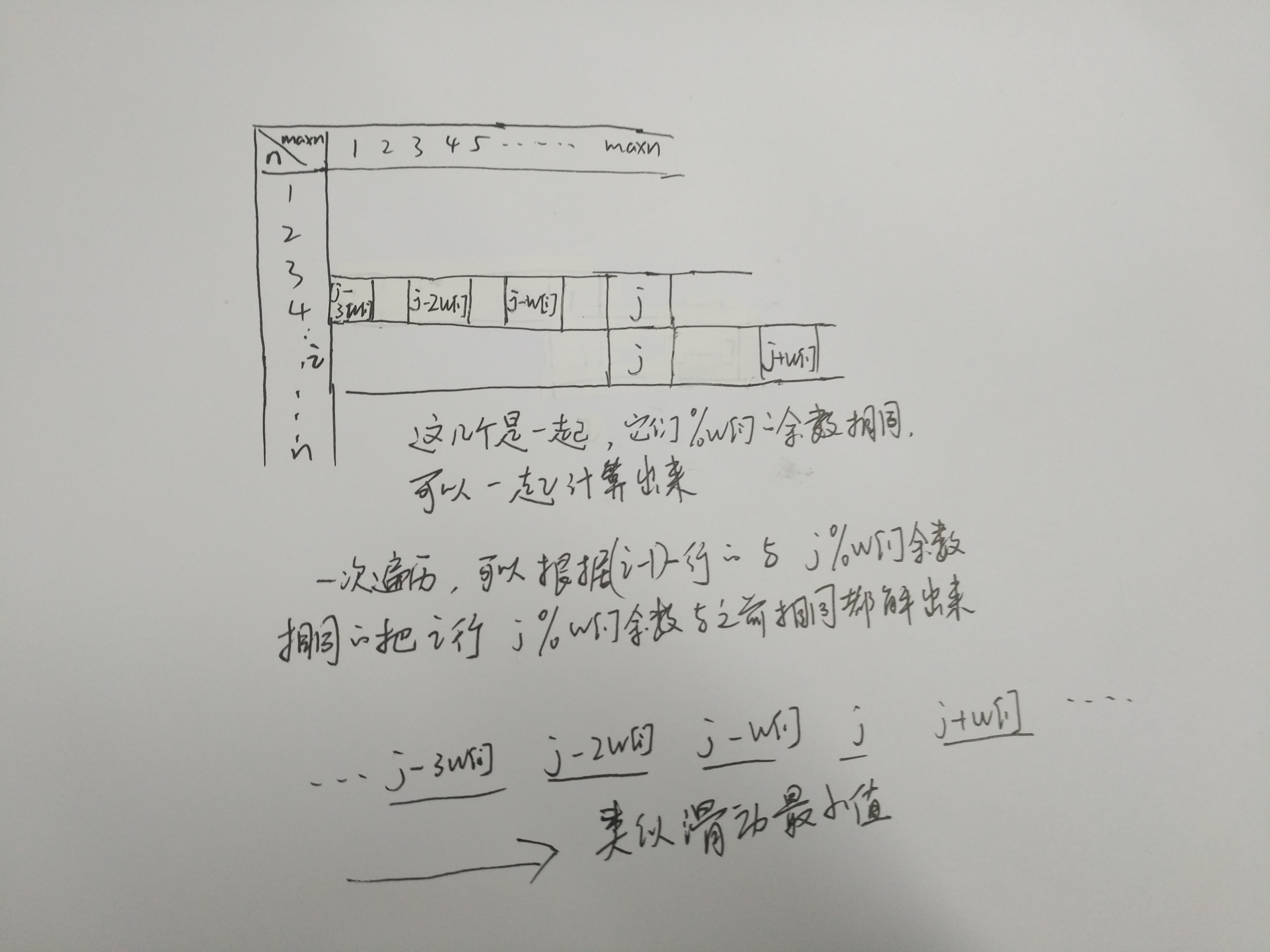## POJ 1276 Cash Machine（单调队列优化多重背包） 转

o
osc_z1hvg4cu

Cash Machine
 Time Limit: 1000MS Memory Limit: 10000K Total Submissions: 38986 Accepted: 14186

Description

A Bank plans to install a machine for cash withdrawal. The machine is able to deliver appropriate @ bills for a requested cash amount. The machine uses exactly N distinct bill denominations, say Dk, k=1,N, and for each denomination Dk the machine has a supply of nk bills. For example,

N=3, n1=10, D1=100, n2=4, D2=50, n3=5, D3=10

means the machine has a supply of 10 bills of @100 each, 4 bills of @50 each, and 5 bills of @10 each.

Call cash the requested amount of cash the machine should deliver and write a program that computes the maximum amount of cash less than or equal to cash that can be effectively delivered according to the available bill supply of the machine.

Notes:
@ is the symbol of the currency delivered by the machine. For instance, @ may stand for dollar, euro, pound etc.

Input

The program input is from standard input. Each data set in the input stands for a particular transaction and has the format:

cash N n1 D1 n2 D2 ... nN DN

where 0 <= cash <= 100000 is the amount of cash requested, 0 <=N <= 10 is the number of bill denominations and 0 <= nk <= 1000 is the number of available bills for the Dk denomination, 1 <= Dk <= 1000, k=1,N. White spaces can occur freely between the numbers in the input. The input data are correct.

Output

For each set of data the program prints the result to the standard output on a separate line as shown in the examples below.

Sample Input

``````735 3  4 125  6 5  3 350
633 4  500 30  6 100  1 5  0 1
735 0
0 3  10 100  10 50  10 10``````

Sample Output

``````735
630
0
0``````

Hint

The first data set designates a transaction where the amount of cash requested is @735. The machine contains 3 bill denominations: 4 bills of @125, 6 bills of @5, and 3 bills of @350. The machine can deliver the exact amount of requested cash.

In the second case the bill supply of the machine does not fit the exact amount of cash requested. The maximum cash that can be delivered is @630. Notice that there can be several possibilities to combine the bills in the machine for matching the delivered cash.

In the third case the machine is empty and no cash is delivered. In the fourth case the amount of cash requested is @0 and, therefore, the machine delivers no cash.

Source

### F[i，v] = max{F[i−1,v−k∗Ci] + k∗Wi |0 ≤ k ≤ Mi}``````#include<iostream>
#include<algorithm>
#include<stack>
#include<cmath>
#include<string>
#include<cstring>
#include<cstdio>
#include<vector>
#include<deque>
using namespace std;
const int mx = 1005;
const int mxw = 1000005;
deque<int>deqv, deq;
int w[mx], m[mx], dp[mxw];

int solve(int maxw, int n)
{
memset(dp, 0, sizeof(dp));
int i, k, j, val;
for (i = 1; i <= n; ++i)
for (j = 0; j < w[i]; j++)///枚举a=j%w[i]
{
while (!deqv.empty())//每枚举一次进行一次单调队列求值
{
deqv.pop_front();
deq.pop_front();
}
for (k = 0; k * w[i] + j <= maxw; k++)
{
val = dp[k * w[i] + j] - k * w[i];///划归为可重复使用的值
while (!deqv.empty() && deqv.back() <= val)///保证deque的队首是最大的
{
deqv.pop_back();
deq.pop_back();
}

deq.push_back(k);
deqv.push_back(val);
dp[k * w[i] + j] =deqv.front() + k * w[i];///从双端队列的头部取出最大值
if (deq.front() == k - m[i])///这一头部已无法使用
{
deq.pop_front();
deqv.pop_front();
}

}
}
return dp[maxw];
}

int main()
{
int maxw, n, i;
while (~scanf("%d%d", &maxw, &n))
{
for (i = 1; i <= n; i++)
scanf("%d%d", &m[i], &w[i]);
printf("%d\n", solve(maxw, n));
}
return 0;
}``````

o

### osc_z1hvg4cu#### 暂无文章

49分钟前
17
0
Android Studio写flutter快捷键

1.stl ：代表StatelessWidget 2.stf ：StatefulWidget 3.cmd + shift + 减号 ：折叠所有代码 4.cmd + 减号 ：折叠当前代码块 5.ctrl + r ：编译运行 6.cmd + s ：hot reload 7.cmd + { :回到...

21
0

ethanleellj

17
0

13
0
Mysql主从同步

1主从同步 1.1Master 1.1.1配置--编辑 my.cnf #编辑 mysql 的 /etc/my.cnf 配置文件vi /etc/my.cnf#添加如下配置server-id=1 #设置服务 IDlog_bin=mysql-bin #启动 binlog...

14
0